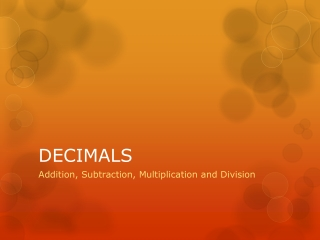DownloadDownload PresentationDECIMALS

# DECIMALS

Télécharger la présentation## DECIMALS

- - - - - - - - - - - - - - - - - - - - - - - - - - - E N D - - - - - - - - - - - - - - - - - - - - - - - - - - -
##### Presentation Transcript

1. DECIMALS Addition, Subtraction, Multiplication and Division

2. 5 5 5 = = = .5 .005 10 1000 .05 100 999 1000 ( 1 + same number of zeros as digits in numerator) D. DECIMAL NUMBERS 1. Decimal System • System of numbers based on ten (10). • Decimal fraction has a denominator of 10, 100, 1000, etc. Written on one line as a whole number, with a period (decimal point) in front. 3 digits .999 is the same as

3. 7 5 is written 5.7 10 Whole Number Decimal Fraction (Tenths) 7 55 is written 55.07 100 Whole Number Decimal Fraction (Hundredths) Decimal Fraction (Tenths) 77 555 is written 555.077 1000 Whole Number Decimal Fraction (Thousandths) Decimal Fraction (Hundredths) Decimal Fraction (Tenths) 2. Reading and Writing Decimals

4. One place .0 tenths Two places .00 hundredths Three places .000 thousandths Four places .0000 ten-thousandths Five places .00000 hundred-thousandths 2. Reading and Writing Decimals (con’t) • Decimals are read to the right of the decimal point. .63 is read as “sixty-three hundredths.” .136 is read as “one hundred thirty-six thousandths.” .5625 is read as “five thousand six hundred twenty-five ten-thousandths.” 3.5 is read “three and five tenths.” • Whole numbers and decimals are abbreviated. 6.625 is spoken as “six, point six two five.”

5. 3. Addition of Decimals • Addition of decimals is same as addition of whole numbers except for the location of the decimal point. Add .865 + 1.3 + 375.006 + 71.1357 + 735 • Align numbers so all decimal points are in a vertical column. • Add each column same as regular addition of whole numbers. • Place decimal point in same column as it appears with each number. .865 1.3 375.006 71.1357 + 735. 0 000 “Add zeros to help eliminate errors.” 0 “Then, add each column.” 0000 1183.3067

6. 4. Subtraction of Decimals • Subtraction of decimals is same as subtraction of whole numbers except for the location of the decimal point. Solve: 62.1251 - 24.102 • Write the numbers so the decimal points are under each other. • Subtract each column same as regular subtraction of whole numbers. • Place decimal point in same column as it appears with each number. 62.1251 - 24.102 “Add zeros to help eliminate errors.” 0 38.0231 “Then, subtract each column.”

7. Decimal point 3 places over. Decimal point 2 places over. 5. Multiplication of Decimals Rules For Multiplying Decimals • Multiply the same as whole numbers. • Count the number of decimal places to the right of the decimal point in both numbers. • Position the decimal point in the answer by starting at the extreme right digit and counting as many places to the left as there are in the total number of decimal places found in both numbers. Solve: 38.639 X 2.08 3 8 .6 3 9 x 2.0 8 3 0 6 9 5 2 “Add zeros to help eliminate errors.” 7 7 2 7 8 0 0 . 8 0 3 4 7 5 2 “Then, add the numbers.” Place decimal point 5 places over from right.

8. Solve: 123.573 137.4 6. Division of Decimals Rules For Dividing Decimals • Place number to be divided (dividend) inside the division box. • Place divisor outside. • Move decimal point in divisor to extreme right. (Becomes whole number) • Move decimal point same number of places in dividend. (NOTE: zeros are added in dividend if it has fewer digits than divisor). • Mark position of decimal point in answer (quotient) directly above decimal point in dividend. • Divide as whole numbers - place each figure in quotient directly above digit involved in dividend. • Add zeros after the decimal point in the dividend if it cannot be divided evenly by the divisor. • Continue division until quotient has as many places as required for the answer.

9. 6. Division of Decimals 9 3 8 9 . 0 137 4 1 2 3 5 7 3 0 . . . . 1 0 9 9 2 1 3 6 5 3 1 2 3 6 6 1 2 8 7 0 1 2 3 6 6 5 0 4 0 4 1 2 2 9 1 8 remainder

10. Decimal Number Practice Exercises “WORK ALL 4 SECTIONS (+, , X, ) 1. Add the following decimals. .6 + 1.3 + 2.8 = 72.8 + 164.02 + 174.01 = 185.7 + 83.02 + 9.013 = 0.93006 + 0.00850 + 3315.06 + 2.0875 = 2. Subtract the following decimals. 2.0666 - 1.3981 = 18.16 - 9.104 = 1.0224 - .9428 = 1.22 - 1.01 = 0.6 - .124 = 18.4 - 18.1 = 1347.008 - 108.134 = 111.010 - 12.163 = 64.7 - 24.0 = Let's check our answers.

11. Decimal Number Practice Exercises 3. Multiply the following decimals. c. 1.6 x 1.6 b. 21.3 x 1.2 3.01 x 6.20 f. 44.02 x 6.01 e. 1.64 x 1.2 d. 83.061 x 2.4 i. 68.14 x 23.6 h. 183.1 x .23 g. 63.12 x 1.12 Let's check our answers.

12. Decimal Number Practice Exercises 4. Divide the following decimals. b. .8 4.6 3000 a. 1.4 4 2.7 0 d. 6 6.6 7 8 6 c. 1.2 6 2 0.4 e. 1.1 110.0 Let's check our answers.